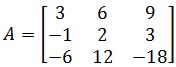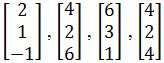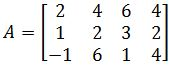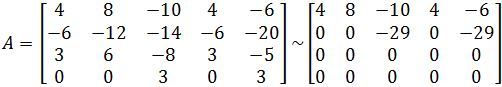# Dimension and rank

### Dimension and rank

#### Lessons

Dimension of a Subspace
The dimension of a non-zero subspace $S$ (usually denoted as dim $S$), is the # of vectors in any basis for $S$. Since the null space and column space is a subspace, we can find their dimensions.

Note: Dimension of the column space = rank

Finding the Rank of a matrix:
1. Find the basis for the column space
2. Count the # of vectors in the basis. That is the rank.
Shortcut: Count the # of pivots in the matrix

Finding the dimensions of the null space:
1. Find the basis for the null space
2. Count the # of vectors in the basis. That is the dimension.
Shortcut: Count the # of free variables in the matrix.

The Rank Theorem
If a matrix $A$ has $n$ columns, then rank $A+$ dim $N(A) = n$.
• Introduction
Dimension and Rank Overview:
a)
Dimension of a subspace
• Dimension = number of vectors in the basis
• Can we find dimension of column space and null space?

b)
Rank of a Matrix
• Find the basis
• Count the # of vectors
• Shortcut = count the # of pivots

c)
Dimension of the Null Space
• Find the general solution
• Put in parametric vector form
• Count the # of vectors
• Shortcut = count the # of free variables

d)
The Rank Theorem
• Rank $A +$ dim $N(A) = n$
• An example of using the theorem

• 1.
Finding the Rank of a matrix
Find the rank of $A$ if:• 2.
Find a basis for the subspace spanned by the given vectors. What is the dimension of the subspace?• 3.
Finding the dimension of the null space
Find the dimension of the null space of $A$ if:• 4.
Utilizing the Rank Theorem
You are given the matrix $A$ and the echelon form of $A$. Find the basis for the column space, and find the rank and the dimensions of the null space.• 5.
Understanding the Theorems
Let the subspace of all solutions of $Ax=0$ have a basis consisting of four vectors, where $A$ is $4 \times 6$. What is the rank of $A$?

• 6.
Let $A$ be a $m \times n$ matrix where the rank of $A$ is $p$. Then what is the dimension of the null space of $A$?

##### Do better in math today
6.
Subspace of $\Bbb{R}^n$
6.1
Properties of subspace
6.2
Column space
6.3
Null space
6.4
Dimension and rank